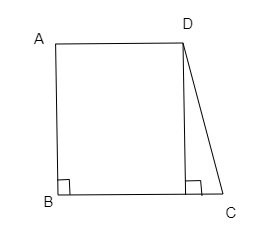# The length of the fence of a trapezium-shaped field $A B C D$ is $130 \mathrm{~m}$ and side $A B$ is perpendicular to each of the parallel sides AD and $B C$. If $B C=54 \mathrm{~m}, C D=19 \mathrm{~m}$ and $A D=42 \mathrm{~m}$, find the area of the field.

Given:

The length of the fence of a trapezium-shaped field $A B C D$ is $130 \mathrm{~m}$ and side $A B$ is perpendicular to each of the parallel sides AD and $B C$.

$B C=54 \mathrm{~m}, C D=19 \mathrm{~m}$ and $A D=42 \mathrm{~m}$.

To do:

We have to find the area of the field.

Solution:

From the figure,$BC=54\ m, CD=19\ m, AD=42\ m$

Perimeter$=$Length of the fence

$130\ m=AB+BC+CD+DA$

$130=AB+54+19+42$

$AB=130-115$

$AB=15\ m$

Area of the field$=\frac{1}{2}\times$Sum of parallel sides $\times$Perpendicular distance(AB)

$=\frac{1}{2}\times(42+54)\times15\ cm^2$

$=\frac{1}{2}\times96\times15\ cm^2$

$=48\times15\ cm^2$

$=720\ cm^2$

Updated on: 10-Oct-2022

22 Views